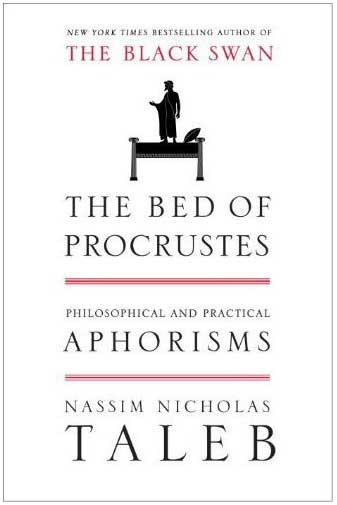## checking for finite variance of importance samplers

Posted in R, Statistics, Travel, University life with tags , , , , , , , , on June 11, 2014 by xi'anOver a welcomed curry yesterday night in Edinburgh I read this 2008 paper by Koopman, Shephard and Creal, testing the assumptions behind importance sampling, which purpose is to check on-line for (in)finite variance in an importance sampler, based on the empirical distribution of the importance weights. To this goal, the authors use the upper tail  of the weights and a limit theorem that provides the limiting distribution as a type of Pareto distribution$\dfrac{1}{\beta}\left(1+\xi z/\beta \right)^{-1-1/\xi}$

over (0,∞). And then implement a series of asymptotic tests like the likelihood ratio, Wald and score tests to assess whether or not the power ξ of the Pareto distribution is below ½. While there is nothing wrong with this approach, which produces a statistically validated diagnosis, I still wonder at the added value from a practical perspective, as raw graphs of the estimation sequence itself should exhibit similar jumps and a similar lack of stabilisation as the ones seen in the various figures of the paper. Alternatively, a few repeated calls to the importance sampler should disclose the poor convergence properties of the sampler, as in the above graph. Where the blue line indicates the true value of the integral.

## bias in estimating bracketed quantile contributions

Posted in Books, R, Statistics, University life with tags , , , , , , on May 16, 2014 by xi'an“Vilfredo Pareto noticed that 80% of the land in Italy belonged to 20% of the population, and vice-versa, thus both giving birth to the power law class of distributions and the popular saying 80/20.”

Yesterday, in “one of those” coincidences, I voluntarily dropped Nassim Taleb’s The Bed of Procrustes in a suburban café as my latest contribution to the book-crossing (or bXing!) concept and spotted a newly arXived paper by Taleb and Douadi. Paper which full title is “On the Biases and Variability in the Estimation of Concentration Using Bracketed Quantile Contributions” and which central idea is that estimating$\kappa_\alpha = \alpha\mathbb{E}[X|X>q_\alpha]\big/\mathbb{E}[X]$

(where qα is the α-level quantile of X) by the ratio$\sum_{i=1}^n \mathbb{I}_{X_i>\hat{q_\alpha}} X_i \big/ \sum_{i=1}^n X_i$

can be strongly biased. And that the fatter the tail (i.e. the lower the power β for a power law tail), the worse the bias. This is definitely correct, if not entirely surprising given that the estimating ratio involves a ratio of estimators, plus an estimator of qα. And that both numerator and denominator have finite variances when the power β is less than 2.  The paper contains a simulation experiment easily reproduced by the following R code

#biased estimator of kappa(.01)
alpha=.01 #tail
omalpha=1-alpha
T=10^4    #simulations
n=10^3    #sample size
beta=1.1  #Pareto parameter
moobeta=-1/beta

kap=rep(0,T)
for (t in 1:T){
sampl=runif(n)^moobeta
quanta=quantile(sampl,omalpha)
kap[t]=sum(sampl[sampl>quanta])/sum(sampl)
}


What is somewhat surprising though is that the paper deems it necessary to run T=10¹² simulations to assess the bias when this bias is already visible in the first digit of κα. Given that the simulation experiment goes as high as n=10⁸, this means the authors simulated 10²⁰ Pareto variables to exhibit a bias a few thousand replicas could have produced. Checking the numerators and denominators in the above collection of ratios also shows that they may take unbelievably large values.)

“…some theories are built based on claims of such increase’ in inequality, as in Piketti (2014), without taking into account the true nature of κ, and promulgating theories about the variation’ of inequality without reference to the stochasticity of the estimation—and the lack of consistency of κ across time and sub-units.”

The more relevant questions about this issue of estimating κα are, in my opinion, (a) why this quantity is of enough practical importance to consider its estimation and to seek estimators that would remain robust as the power β varies arbitrarily close to 1; (b) in which sense there is anything more to the phenomenon than the difficulty in estimating β itself;  and (c) what is the efficient asymptotic variance for estimating κα (since there is no particular reason to only consider the most natural estimator). Despite the above quote, that the paper constitutes  a major refutation of Piketty’s Capital in the Twenty-First Century is rather unlikely!